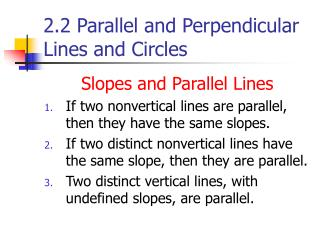DownloadDownload Presentation2.2 Parallel and Perpendicular Lines and Circles

# 2.2 Parallel and Perpendicular Lines and Circles

Télécharger la présentation## 2.2 Parallel and Perpendicular Lines and Circles

- - - - - - - - - - - - - - - - - - - - - - - - - - - E N D - - - - - - - - - - - - - - - - - - - - - - - - - - -
##### Presentation Transcript

1. 2.2 Parallel and Perpendicular Lines and Circles Slopes and Parallel Lines • If two nonvertical lines are parallel, then they have the same slopes. • If two distinct nonvertical lines have the same slope, then they are parallel. • Two distinct vertical lines, with undefined slopes, are parallel.

2. Solution X1=-2 Y1=-7

3. What is the slope of the line? Given equation Slope of the line is –5.

4. X1=-2, y1=-7, and m=-5

5. Practice Exercise

6. Answer to the Practice Exercise

7. Slopes and Perpendicular Lines • If two nonvertical lines are perpendicular, then the product of their slopes is –1. • If the product of the slopes of two lines is –1, then lines are perpendicular. • A horizontal line having zero slope is perpendicular to a vertical line having undefined slope.

8. Example 2: Finding the Slope of a Line Perpendicular to a

9. Solution Solve the given equation for y. Slope is –3/2.

10. Given line has slope –3/2.

11. Practice Exercise

13. Definition of a Circle A circle is the set of all points in a plane that are equidistant from a fixed point called the center. The fixed distance from the circle’s center to any point on the circle is called the radius.

14. The Standard Form of the Equation of a Circle Center Any point on the circle

15. Solution

16. Practice Exercises

18. Example 4: Using the Standard Form of a Circle’s

19. Solution

20. Practice Exercise